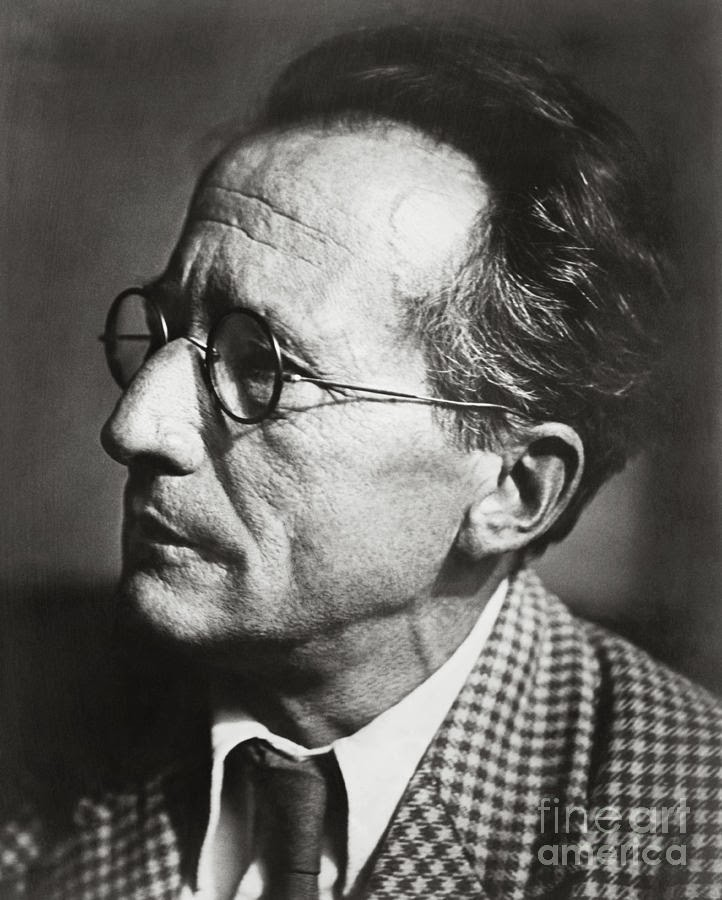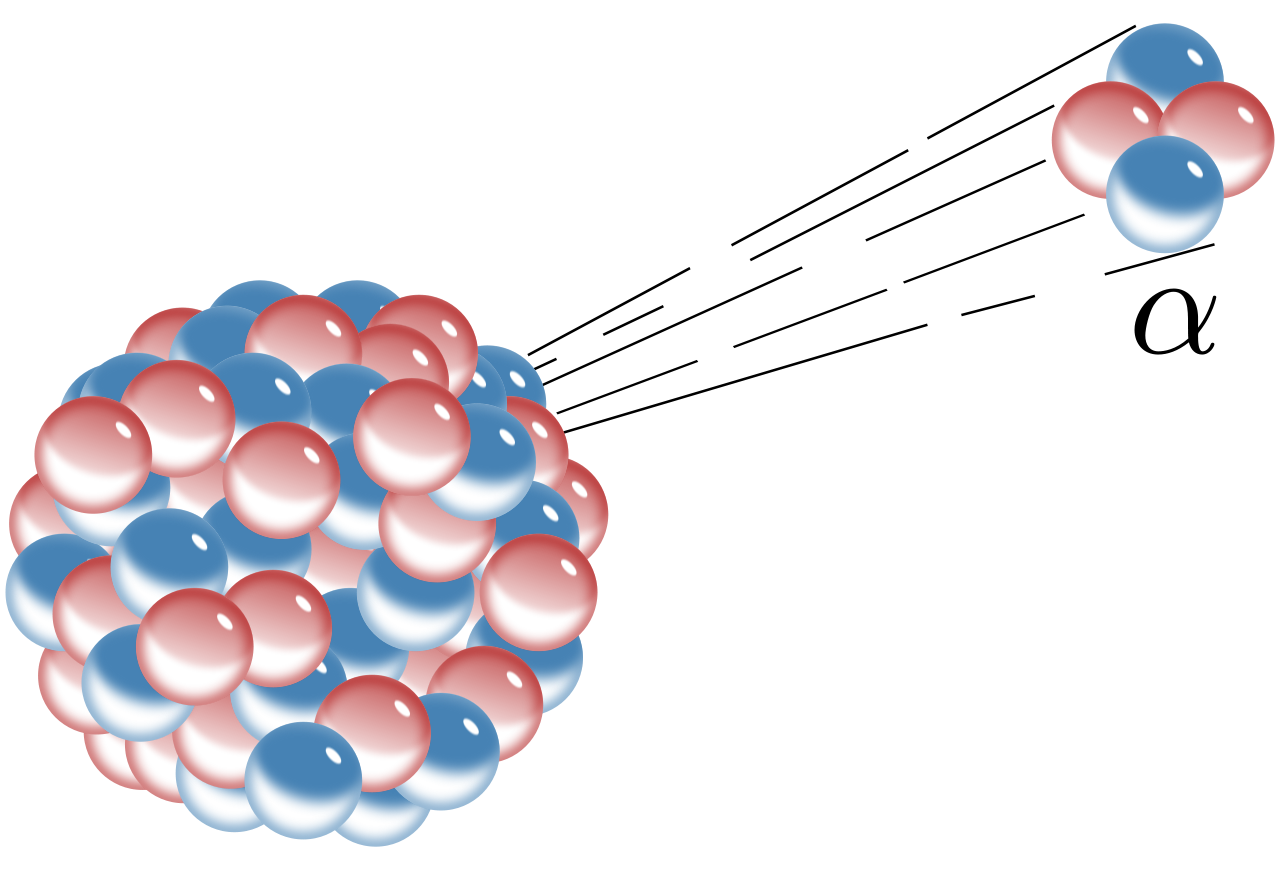## Thursday, 21 August 2014

### What is Quantum Tunneling?Erwin Schrodinger Source: http://fineartamerica.com/
by Sparshita Dey

Quantum Tunneling is a quantum phenomenon where particles with less energy than that of a potential barrier can still cross the energy barrier, by “tunneling” through. This means that the particle, which according to classical mechanics, cannot possibly end up on the other side of the barrier, has a chance of being found within or on the other side of the barrier – corresponding to a finite probability of being found in these “classically forbidden regions”. This will be explained in a more simplistic manner later on.

In this article, I will aim to explain how some concepts in quantum mechanics means that tunneling is possible. This will involve having a look at various examples of tunneling phenomena, what wave functions are and also the time-independent Schrodinger Equation.

As with any unfamiliar concept, I find that the best place to start would be with an analogy featuring objects we are very familiar with in everyday life.

So imagine you and your friend standing either side of a solid wall.

Neither you nor your friend can see each other through or over the wall.
Your friend now throws a tennis ball at the wall.

In this case, it’s obvious that the ball will fly through the air until it reaches the wall and then bounce back towards your friend. This is the view we are all familiar with: the classical view.

Now imagine if this did not happen. Imagine if instead, the ball, on reaching the wall, appears to disappear into the wall (according to your friend) and then appears to be coming at you (on the other side of the wall).

This is the view put forward by quantum mechanics, a view deemed impossible by classical mechanics. The ball seems to have “tunneled” through the wall! This is therefore known as Quantum Tunneling.

I think it is safe to say that no one has ever experienced throwing a ball at a wall and seeing it miraculously appear from the other side of it. However, following the analogy, if we relate the tennis ball to an electron, and the wall to a potential/energy barrier, we see that there is actually evidence of electrons being able to tunnel through potential barriers to cross over to the other side.

Alpha Decay
A more realistic example where tunneling plays a significant role is in alpha decay, where an unstable nucleus releases an alpha particle (a Helium nucleus with 2 neutrons and 2 protons). In this situation there are two forces we need to consider: the Coulomb force (repulsion of two positive charges) and the strong nuclear force

The Coulomb force arises due to Coulomb’s law, which states “any two point charges exert an electrical force on each other that is proportional to the product of their charges and inversely proportional to the square of the distance between them”. As the alpha particle has a charge of 2+ due to having two positively charged protons and the nucleus of an atom is also positively charged, this force must be an electrostatic repulsion.Source: en.wikipedia.org
The strong nuclear force is the strong (but short-ranged) attractive force, which acts within the nucleus between hadrons (protons and neutrons) keeping them together.

We can now see that the Coulomb force acts to “help” the alpha particles to escape from the nucleus, while the strong force (which is stronger than the repulsion) acts to keep the alpha particles within the nucleus. Therefore, the alpha particles, with less energy than required to overcome the nuclear potential barrier, should not be able to escape the nucleus following the classical laws. However, quantum mechanics also predicts wave-particle duality (so a particle can act as both a wave and a particle) meaning that if the alpha particle were to be a wave (its behavior modeled by a wave function), there would be a finite probability of the alpha particle overcoming the potential barrier and escaping. As we all know that alpha decay does occur and a Geiger counter definitely clicks when placed in front of a source, which decays to release alpha particles (can be tested by observing beams in electric/magnetic fields), there is nothing to say that this explanation is false.

From this example we see that the wave characteristic of a particle must be very important as this allows there to be a probability that a particle can tunnel through a potential barrier. Due to this property, a quantum mechanical system / a particle e.g. an electron or an alpha particle can be completely described as a wave function ψ(x,t) - which is a single-valued function of position.  This wave function has the characteristic that when squared, it gives the probability density P(x) (the probability per unit length) of finding a particle at position x. This is shown graphically:

From GCSE knowledge we know the area underneath a histogram with y-axis frequency density gives frequency, similar the area under the graph with y-axis probability density must give probability. This can also be represented by the integral
As we know that probabilities of all outcomes must add to make 1, one further characteristic of our wave function must be that:

But how does the wave function help us to work out the probability of tunneling?

This is where we need the Time-independent Schrodinger equation:

which is in the form

Which according to Wikipedia means: “When the Hamiltonian operator acts on a certain wave function Ψ, and the result is proportional to the same wave function Ψ, then Ψ is a stationary state, and the proportionality constant, E, is the energy of the state Ψ.”

The function ψ is thus known as an Eigen function where E is known as the Eigen value – this shows how E can only take specific/discrete values i.e. the Schrodinger Equation gives quantized energies of the system.

The Hamiltonian operator is defined as:

It operates on the energy E of a particle = kinetic energy + potential energy of the particle.

In order to satisfy the time-independent Schrodinger equation we know that the solution ψ may only take certain values as it is an Eigen equation. The specific solution for the above has been calculated to be in the form of ψ =   Ae±ax (similar to the wave function of a plane wave).

Using this, we can now investigate whether or not there is a probability of tunneling for an electron trying to cross a potential barrier:

So the energy gap between that of the particle and the barrier is given by E – V0

Meaning that the equation we are trying to solve looks like:

We also know that the solution is of the form ψ =   Ae±ax in this case negative because the amplitude A of the wave will have to decrease after tunneling (exponential decay). However, we don’t know what the constant “a” is. Hence, to calculate this, we can substitute the wave function into the Schrodinger equation and rearrange/ simplify:

where E is the energy of the particle in Joules
m is the mass of the particle in Kg
V0 is the height of the potential barrier in Joules
d is the thickness of the barrier in m

The ratio of the incident to exit amplitude of the particle as a wave is e-ax where a is as calculated above.

The probability of a the particle having tunneled through the barrier is proportional to amplitude squared.
Therefore the probability of finding that the particle has tunneled through is (e-ax )2

For example:
if E = 5 eV = (0.80 x 10-18 ) (to 2 s.f.)

m = 9.11 x 10-31

V0 = 6 eV = (0.96 x 10-18) (to 2 s.f.)

d = 0.5 x 10-9

Then a = 0.51 x 1010
(just by substituting into our previously calculated expression for a)
So the ratio e-ax 0.77 x 10-1

And therefore as Probability is proportional to Amplitude2
The ratio squared gives the probability = 0.60 x 10-2

Therefore we have finally shown that the probability of a particle like an electron tunneling in a scenario is finite and non-zero.Source: www.gosunstove.com

Overall, tunneling is not just a phenomenon, which applies in a few isolated cases here and there. Tunneling is also very important in computers/ circuits – objects we use everyday. However, most importantly (arguably), tunneling allows fusion to take place in our Sun - as although the Sun may be very hot, the hydrogen atoms still don’t have enough energy to fuse. So essentially tunneling allows the Sun to burn its fuel and release its energy as light, without which there would be no life on Earth.

References:
www.youtube.com - What is Quantum Tunneling?
YouTube Quantum Tunneling – Minute Physics
YouTube How Quantum Tunneling works by Ivar Giaever
Wikipedia – Quantum Tunneling
Wikipedia – Heisenberg Uncertainty Principle
Wikipedia – Wave function
Wikipedia – Schrodinger Equation
A Cavendish Quantum Mechanics Primer – M. Warner and A.C.H. Cheung
http://www.physicsoftheuniverse.com/topics_quantum_uncertainty.html

1.I absolutely love this - especially your diagrams ;) I recently used it to explain a bit about the quantum world to my friends!

2.Thank you! Glad you found it useful :D

Hi there!

We'd love it if you'd share your thoughts and ideas. Don't forget to check back after commenting because we try to reply to all of your comments.

Just remember to be nice, please!

:)